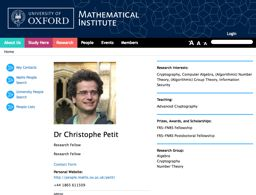# Recent advances in Elliptic Curve Discrete Logarithm algorithms03/30/2016
Speaker(s) : Christophe PETIT (University of Oxford)
The elliptic curve discrete logarithm problem (ECDLP) is one of the core number theory problems used in cryptography today, for example in TLS protocol. The elliptic curve discrete logarithm problem is believed to be much harder than the discrete logarithm problem over finite fields and the factorization problem, as the best attacks for commonly used parameters are still generic DLP algorithms. As key sizes in applications are chosen accordingly, it is important to understand the exact hardness of ECDLP.
In this talk, I will review recent advances in solving this problem using index calculus algorithms, starting from the work of Semaev in 2004. As it happens, we now have subexponential (in L(2/3) time) algorithms for special families of parameters, but these parameters are however not really used in practice. I will then show how these algorithms can potentially be adapted to elliptic curves defined over binary fields of prime degree extensions and to elliptic curve defined over prime fields (the two families that appear in standards and applications), and I will describe remaining challenges in improving both their complexity and their analysis.

Cecile.Pierrot (at) nulllip6.fr